## GATE 2006-IT

 Question 1

In a certain town, the probability that it will rain in the afternoon is known to be 0.6. Moreover, meteorological data indicates that if the temperature at noon is less than or equal to 25°C, the probability that it will rain in the afternoon is 0.4. The temperature at noon is equally likely to be above 25°C, or at/below 25°C. What is the probability that it will rain in the afternoon on a day when the temperature at noon is above 25°C?

 A 0.4 B 0.6 C 0.8 D 0.9
Engineering-Mathematics       Probability
Question 1 Explanation:
Probability rain in afternoon = (0.5×probability of rain when temp≤25) + (0.5×Probability of rain when temp>25)
0.6 = (0.5×0.4) + (0.5×P(rain at temp>25)
0.6 = (2) + (0.5×P(rain at temp>25)
P(rain at temp>25) = 0.8
 Question 2

For the set N of natural numbers and a binary operation f : N x N → N, an element z ∊ N is called an identity for f, if f (a, z) = a = f(z, a), for all a ∊ N. Which of the following binary operations have an identity?

1. f(x,y) = x + y - 3
2. f(x,y) = max(x, y)
3. f(x,y) = xy
 A 1 and 2 only B 2 and 3 only C 1 and 3 only D None of these
Engineering-Mathematics       Sets and Relations
Question 2 Explanation:
(1) f(x, y) = x + y -3
y = 3
Here, identity element is 3.
(2) f(x, y) = max(x, y) = x = max(y, x)
⇒ y = 1
Here, identity element = 1
(3) f(x, y) = xny is not equal to f(y, x) = ynx
So, no identity element.
 Question 3

In the automaton below, s is the start state and t is the only final state.Consider the strings u = abbaba, v = bab, and w = aabb. Which of the following statements is true?

 A The automaton accepts u and v but not w B The automaton accepts each of u, v, and w C The automaton rejects each of u, v, and w D The automaton accepts u but rejects v and w
Theory-of-Computation       Finite-Automata
Question 3 Explanation:
(i) u = abbabawhere t is final state
(ii) v = bab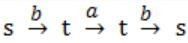s is not final state
(iii) w = aabbs is not final state
 Question 4

In the context-free grammar below, S is the start symbol, a and b are terminals, and ϵ denotes the empty string

`S → aSa | bSb | a | b | ϵ `
Which of the following strings is NOT generated by the grammar?

 A aaaa B baba C abba D babaaabab
Theory-of-Computation       Contest-Free-Grammar
Question 4 Explanation:
S → aSa | bSb | a | b | ϵ
Given string accepts all palindromes.
Option B → baba is not palindrome.
So, this is not accepted by S.
 Question 5

Which regular expression best describes the language accepted by the non-deterministic automaton below?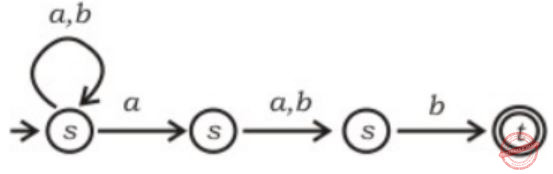A (a + b)* a(a + b)b B (abb)* C (a + b)* a(a + b)* b(a + b)* D (a + b)*
Theory-of-Computation       Regular-Expressions
Question 5 Explanation:Question 6

Given a Boolean function f (x1, x2, …, xn), which of the following equations is NOT true.

 A f (x1, x2, …, xn) = x1’f(x1, x2, …, xn) + x1f(x1, x2, …, xn) B f (x1, x2, …, xn) = x2f(x1, x2, …, xn) + x2’f(x1, x2, …, xn) C f (x1, x2, …, xn) = xn’f(x1, x2, …, 0) + xnf(x1, x2, …,1) D f (x1, x2, …, xn) = f(0, x2, …, xn) + f(1, x2, .., xn)
Engineering-Mathematics       Sets-And-Relation
Question 6 Explanation:
Proceed by taking f(x1) = x,
LHS: f(x1) = 0 where x1 = 0
LHS: f(x1) = 1 when x1 = 1
RHS: f(0) + f(1) = 0 + 1 = always 1
 Question 7

The addition of 4-bit, two’s complement, binary numbers 1101 and 0100 results in

 A 0001 and an overflow B 1001 and no overflow C 0001 and no overflow D 1001 and an overflow
Digital-Logic-Design       Number-Systems
Question 7 Explanation:
2's complement of 1101 = 0011
2's complement of 1100 = 1100
Now convert 1111 to normal form.
⇒ 0000 (1's complement)
⇒ 0001 (2's complement) No carry bit.
 Question 8

Which of the following DMA transfer modes and interrupt handling mechanisms will enable the highest I/O band-width?

 A Transparent DMA and Polling interrupts B Cycle-stealing and Vectored interrupts C Block transfer and Vectored interrupts D Block transfer and Polling interrupts
Computer-Organization       Modes-of-Transfer
Question 8 Explanation:
→ CPU has highest bandwidth in transparent DMA and polling. But it asked for I/O bandwidth not CPU bandwidth option. A is wrong.
→ In case of cycle stealing in each cycle time derive send data then wait again few CPU cycle it sends to memory. So, option B is wrong.
→ In case of polling CPU takes the initiative. So I/O bandwidth cannot be high. So, option D is wrong.
→ Consider block transfer in each single block device. Send data so bandwidth must be high. This makes option (C) correct.
 Question 9

In a binary tree, the number of internal nodes of degree 1 is 5, and the number of internal nodes of degree 2 is 10. The number of leaf nodes in the binary tree is

 A 10 B 11 C 12 D 15
Data-Structures       Binary-Trees
Question 9 Explanation:
A node in a binary tree has degree 0, 1, 2.
No. of 1 degree nodes = 5
No. of 2 degree nodes = 10
Total no. of edges = (1*5) + (2*10) = 5 + 20 = 25
So, Total no. of edges = 25 + 1 = 26 (No. of nodes in a tree is 1 more than no. of edges)
Total no. of leaf nodes (node with 0 degree) = 26 - 5 - 10 = 11
 Question 10

A problem in NP is NP-complete if

 A It can be reduced to the 3-SAT problem in polynomial time B The 3-SAT problem can be reduced to it in polynomial time C It can be reduced to any other problem in NP in polynomial time D Some problem in NP can be reduced to it in polynomial time
Algorithms        P-NP
Question 10 Explanation:
A problem is in NP becomes NP-complete if all NP problems can be reduced to it in polynomial time. This is same as reducing any of the NPC problem to it. 3SAT being an NPC problem reducing it to a NP problem would mean that NP problem is NPC.
 Question 11

If all the edge weights of an undirected graph are positive, then any subset of edges that connects all the vertices and has minimum total weight is a

 A Hamiltonian cycle B grid C hypercube D tree
Data-Structures       Tree
Question 11 Explanation:
MST:
If all edge weights of an undirected graph are positive, then any subset of edges that connects all the vertices and has minimum total weight is minimum spanning tree.
 Question 12

In the working-set strategy, which of the following is done by the operating system to prevent thrashing?
1. It initiates another process if there are enough extra frames.
2. It selects a process to suspend if the sum of the sizes of the working-sets exceeds the total number of available frames.

 A 1 only B 2 only C Neither 1 nor 2 D Both 1 and 2
Operating-Systems       Thrashing
Question 12 Explanation:
According to the concept of thrashing both statement (1) and (2) are true.
 Question 13

The process state transition diagram of an operating system is as given below.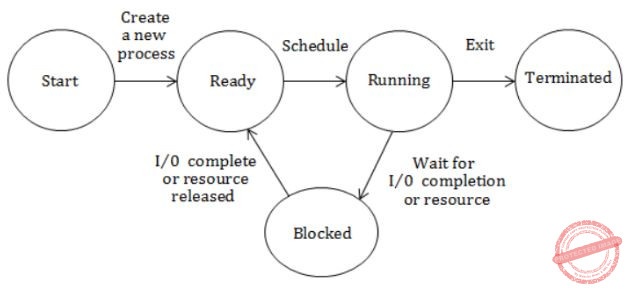Which of the following must be FALSE about the above operating system?

 A It is a multiprogrammed operating system B It uses preemptive scheduling C It uses non-preemptive scheduling D It is a multi-user operating system
Operating-Systems       Process-Scheduling
Question 13 Explanation:
Option B is wrong. Because given OS is non-preempting scheduling algorithm.
 Question 14

Consider the relations r1(P, Q, R) and r2(R, S, T) with primary keys P and R respectively. The relation r1 contains 2000 tuples and r2 contains 2500 tuples. The maximum size of the join r1⋈ r2 is :

 A 2000 B 2500 C 4500 D 5000
Database-Management-System       Relational-Algebra
Question 14 Explanation:
r1⋈ r2 is a join operation done on the common attribute R. So this can have 2000 tuples.
 Question 15

Which of the following relational query languages have the same expressive power?
1. Relational algebra
2. Tuple relational calculus restricted to safe expressions
3. Domain relational calculus restricted to safe expressions

 A 2 and 3 only B 1 and 2 only C 1 and 3 only D 1, 2 and 3
Database-Management-System       Relational-Query-Languages
Question 15 Explanation:
Given all three languages have same expressive power.
 Question 16

The cyclomatic complexity of the flow graph of a program provides

 A an upper bound for the number of tests that must be conducted to ensure that all statements have been executed at most once B a lower bound for the number of tests that must be conducted to ensure that all statements have been executed at most once C an upper bound for the number of tests that must be conducted to ensure that all statements have been executed at least once D a lower bound for the number of tests that must be conducted to ensure that all statements have been executed at least once
Question 16 Explanation:
Note: Out of syllabus.
 Question 17

With respect to software testing, consider a flow graph G with one connected component. Let E be the number of edges, N be the number of nodes, and P be the number of predicate nodes of G. Consider the following four expressions:
1. E - N + P
2. E - N + 2
3. P + 2
4. P + 1
The cyclomatic complexity of G is given by

 A 1 or 3 B 2 or 3 C 2 or 4 D 1 or 4
Question 17 Explanation:
Note: Out of syllabus.
 Question 18

HELO and PORT, respectively, are commands from the protocols

 A FTP and HTTP B TELNET and POP3 C HTTP and TELNET D SMTP and FTP
Computer-Networks       Network-Protocols
Question 18 Explanation:
Note: Out of syllabus.
 Question 19

Which of the following statements is TRUE?

 A Both Ethernet frame and IP packet include checksum fields B Ethernet frame includes a checksum field and IP packet includes a CRC field C Ethernet frame includes a CRC field and IP packet includes a checksum field D Both Ethernet frame and IP packet include CRC fields
Computer-Networks       Ethernet
Question 19 Explanation:
Ethernet frame:IP packet:IP Datagram:Question 20

Which of the following statement(s) is TRUE?
1. A hash function takes a message of arbitrary length and generates a fixed length code.
2. A hash function takes a message of fixed length and generates a code of variable length.
3. A hash function may give the same hash value for distinct messages.

 A 1 only B 2 and 3 only C 1 and 3 only D 2 only
Computer-Networks       Network-Security
Question 20 Explanation:
(1) A hash function takes a message of arbitrary length and generates a fixed length code. So this is correct.
(2) Statement-2 is wrong, refer statement-1.
(3) Statement-3 is correct, for example hash function N%10, this will generate same values for 1 as well as 2!
 Question 21

Consider the following first order logic formula in which R is a binary relation symbol.

` ∀x∀y (R(x, y)  => R(y, x)) `
The formula is

 A satisfiable and valid B satisfiable and so is its negation C unsatisfiable but its negation is valid D satisfiable but its negation is unsatisfiable
Engineering-Mathematics       Propositional-Logic
Question 21 Explanation:
The given relation is known to be symmetry. We have both symmetric relations possible as well as antisymmetric but neither always holds for all sets. So they both are valid but are satisfiable.
 Question 22

When a coin is tossed, the probability of getting a Head is p, 0 < p < 1. Let N be the random variable denoting the number of tosses till the first Head appears, including the toss where the Head appears. Assuming that successive tosses are independent, the expected value of N is

 A 1/p B 1/(1-p) C 1/p2 D 1/(1-p2)
Engineering-Mathematics       Probability
Question 22 Explanation:
E = 1 × P + (2 × (1 - p) p) + (3 × ( 1 - p) (1 - p) p) + ......
Multiply both sides with (1 - p) and subtract,
E - (1 - p) E = 1 × p + (1 - p) p + (1 - p) (1 - p) p + ......
E - (1 - p) E = p/(1 - (1 - p))
(1 - 1 + p) E = 1
pE = 1
E = 1/p
 Question 23

Let P, Q and R be sets let Δ denote the symmetric difference operator defined as PΔQ = (P U Q) - (P ∩ Q). Using Venn diagrams, determine which of the following is/are TRUE?
1. PΔ (Q ∩ R) = (P Δ Q) ∩ (P Δ R)
2. P ∩ (Q ∩ R) = (P ∩ Q) Δ (P Δ R)

 A 1 only B 2 only C Neither 1 nor 2 D Both 1 and 2
Engineering-Mathematics       Sets-And-Relation
Question 23 Explanation: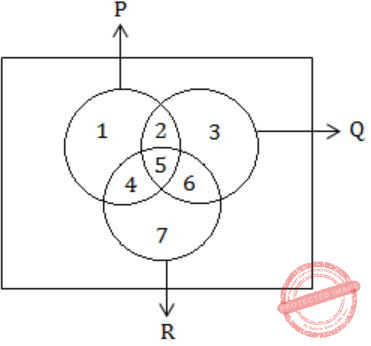P = {1, 2, 4, 5}
Q = {2, 3, 5, 6}
R = {4, 5, 6, 7}
(1) P Δ (Q ∩ R) = (P Δ Q) ∩ (P Δ R)
P Δ ({2,3,5,6} ∩ {4,5,6,7}) = ({1,2,4,5} Δ {2,3,5,6} ∩ {1,2,4,5} Δ {4,5,6,7})
P Δ {5,6} = ({1,2,3,4,5,6} - {2,5}) ∩ ({1,2,4,5,6,7} - {4,5})
({1,2,4,5} Δ {5,6}) = {1,3,4,6} ∩ {1,2,6,7}
{1,2,4,5,6} - {5} = {1,6}
{1,2,4,6} ≠ {1,6}
Statement-1 is False.
(2) P ∩ (Q ∩ R) = (P ∩ Q) Δ (P Δ R)
LHS:
{1,2,4,5} ∩ {5,6} = {5}
RHS:
({1,2,4,5} ∩ {2,3,5,6}) Δ ({1,2,4,5} Δ {4,5,6,7})
{2,5} Δ ({1,2,4,5,6,7} - {4,5})
{2,5} Δ {1,2,6,7}
{1,2,5,6,7} - {2}
{1,5,6,7}
LHS ≠ RHS
Statement - 2 is also wrong.
 Question 24

What is the cardinality of the set of integers X defined below?
X = {n | 1 ≤ n ≤ 123, n is not divisible by either 2, 3 or 5}

 A 28 B 33 C 37 D 44
Engineering-Mathematics       Sets
Question 24 Explanation:
n = 123
A = set of numbers divisible by 2
B = set of numbers divisible by 3
C = set of numbers divisible by 5
n(A∪B∪C) = n(A) + n(B) + n(C) - n(A∩B) - n(B∩C) - n(C∩A) + n(A∩B∩C)
= ⌊123/2⌋ + ⌊123/3⌋ + ⌊123/5⌋ - ⌊123/6⌋ - ⌊123/15⌋ - ⌊123/10⌋ + ⌊123/30⌋
= 61 + 41 + 24 - 20 -12 - 8 + 4
= 90
Total no. that are not divisible
= n - n(A∪B∪C)
= 123 - 90
= 33
 Question 25

Consider the undirected graph G defined as follows. The vertices of G are bit strings of length n. We have an edge between vertex u and vertex v if and only if u and v differ in exactly one bit position (in other words, v can be obtained from u by flipping a single bit). The ratio of the chromatic number of G to the diameter of G is

 A 1/(2n-1) B 1/n C 2/n D 3/n
Engineering-Mathematics       Graph-Theory
Question 25 Explanation:
For the given condition we can simply design a K-map and mark an edge between every two adjacent cells in K-map.
That will give us a bipartite graph, with chromatic number = 2
Also from the same we can conclude that we need for a 'n' bit string, to traverse no more than (n-1) edges or 'n' vertices to get a path between two arbitrary points. So the ratio is (2/n).
 Question 26

What are the eigenvalues of the matrix P given belowAB a, a, a C 0, a, 2a D -a, 2a, 2a
Engineering-Mathematics       Linear-Algebra
Question 26 Explanation:Question 27

Match the following iterative methods for solving algebraic equations and their orders of convergence.A 1-R, 2-S, 3-P, 4-Q B 1-S, 2-R, 3-Q, 4-P C 1-S, 2-Q, 3-R, 4-P D 1-S, 2-P, 3-Q, 4-R
Engineering-Mathematics       Match-the-Following
Question 27 Explanation:
Note: Out of syllabus.
 Question 28

The following definite integral evaluates to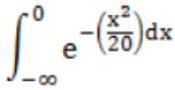A 1/2 B π √10 C √10 D π E None of the above
Engineering-Mathematics       Calculus
Question 28 Explanation:Question 29

Consider the regular grammar below

```S → bS | aA | ϵ
A → aS | bA ```

The Myhill-Nerode equivalence classes for the language generated by the grammar are

 A {w ∈ (a + b)* | #a(w) is even) and {w ∈ (a + b)* | #a(w) is odd} B {w ∈ (a + b)* | #a(w) is even) and {w ∈ (a + b)* | #b(w) is odd} C {w ∈ (a + b)* | #a(w) = #b(w) and {w ∈ (a + b)* | #a(w) ≠ #b(w)} D {ϵ}, {wa | w ∈ (a + b)* and {wb | w ∈ (a + b)*}
Theory-of-Computation       Regular-Grammar
Question 29 Explanation: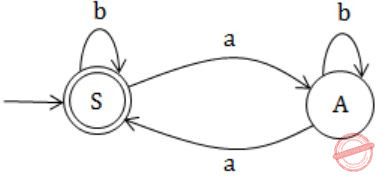⇒ This results even number, no. of a's.
 Question 30

Which of the following statements about regular languages is NOT true?

 A Every language has a regular superset B Every language has a regular subset C Every subset of a regular language is regular D Every subset of a finite language is regular
Theory-of-Computation       Regular Languages
Question 30 Explanation:
Regular languages are not closed under subset.
 Question 31

Which of the following languages is accepted by a non-deterministic pushdown automaton (PDA) but NOT by a deterministic PDA?

 A {anbncn ∣ n≥0} B {albmcn ∣ l≠m or m≠n} C {anbn ∣ n≥0} D {ambn∣ m,n≥0}
Theory-of-Computation       Push-Down-Automata
Question 31 Explanation:
At a time, the DPDA can compare 'a' and 'b' or 'b' and 'c' but not both.
To compare both conditions at the same time, we need a NPDA.
 Question 32

Let L be a context-free language and M a regular language. Then the language L ∩ M is

 A always regular B never regular C always a deterministic context-free language D always a context-free language
Theory-of-Computation       Identify-Class-Language
Question 32 Explanation:
CFL is closed under regular intersection.
 Question 33

Consider the pushdown automaton (PDA) below which runs over the input alphabet (a, b, c). It has the stack alphabet {Z0, X} where Z0 is the bottom-of-stack marker. The set of states of the PDA is (s, t, u, f} where s is the start state and f is the final state. The PDA accepts by final state. The transitions of the PDA given below are depicted in a standard manner. For example, the transition (s, b, X) → (t, XZ0) means that if the PDA is in state s and the symbol on the top of the stack is X, then it can read b from the input and move to state t after popping the top of stack and pushing the symbols Z0 and X (in that order) on the stack.

```(s, a, Z0) → (s, XXZ0)
(s, ϵ, Z0) → (f, ϵ)
(s, a, X) → (s, XXX)
(s, b, X) → (t, ϵ)
(t, b, X) → (t,.ϵ)
(t, c, X) → (u, ϵ)
(u, c, X) → (u, ϵ)
(u, ϵ, Z0) → (f, ϵ) ```

The language accepted by the PDA is

 A {albmcn | l = m = n} B {albmcn | l = m} C {albmcn | 2l = m+n} D {albmcn | m=n}
Theory-of-Computation       Push-Down-Automata
Question 33 Explanation:For every 'a' we put two X in stacks [at state S].
After that for every 'b' we pop out one X [reach to state t].
After that for every 'c' we pop out one X [reach to state u].
If all X are popped out then reached to final state f, means for every 'b' and 'c' there is 'a'. 'a' is followed by 'b' and 'b' is followed by 'c'.
Means,
Sum of no. of b's and no. of c's = twice of no. of a's
i.e., {albmcn | 2l = m+n}
 Question 34

In the context-free grammar below, S is the start symbol, a and b are terminals, and ϵ denotes the empty string.

```S → aSAb | ϵ
A → bA | ϵ ```

The grammar generates the language

 A ((a + b)* b)* B {ambn | m ≤ n} C {ambn | m = n} D a* b*
Theory-of-Computation       Contest-Free-Grammar
Question 34 Explanation:
Option A:
((a + b)* b)* → It accepts string aa but given grammar does not accepts.
Option C&D:
→ abb accepted by given grammar but option C & D are not accepting.
 Question 35

The boolean function for a combinational circuit with four inputs is represented by the following Karnaugh map.Which of the product terms given below is an essential prime implicant of the function?

 A QRS B PQS C PQ'S' D Q'S'
Digital-Logic-Design       K-Map
Question 35 Explanation:
Essential prime implicants which are grouped only by only one method or way. So, in the given question corner's ones are grouped by only one method.
 Question 36

The majority function is a Boolean function f(x, y, z) that takes the value 1 whenever a majority of the variables x, y, z and 1. In the circuit diagram for the majority function shown below, the logic gates for the boxes labeled P and Q are, respectively,A XOR, AND B XOR, XOR C OR, OR D OR, AND
Digital-Logic-Design       Boolean-Functions
Question 36 Explanation:Thus we have OR and AND which gives different outputs on (0,0) and (1,1).
The encodes can be hence select from the two and decide output of the function according to x.
 Question 37

For a state machine with the following state diagram the expression for the next state S+ in terms of the current state S and the input variables x and y isA S+ = S’ . y’ + S . x B S+ = S. x . y’ + S’ . y . x’ C S+ = x . y’ D S+ = S’ . y + S . x’col
Theory-of-Computation       Finite-Automata
Question 37 Explanation: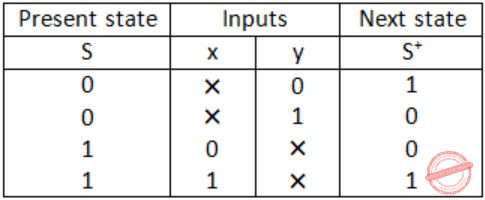From the table:
S' = S'y' + Sx
 Question 38

When multiplicand Y is multiplied by multiplier X = xn-1xn-2 ...x0 using bit-pair recoding in Booth's algorithm, partial products are generated according to the following table.The partial products for rows 5 and 8 are

 A 2Y and Y B -2Y and 2Y C -2Y and 0 D 0 and Y
Digital-Logic-Design       Number-Systems
Question 38 Explanation: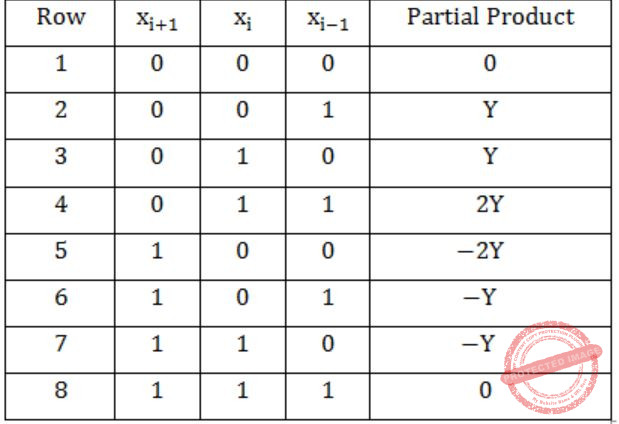⇒ -2Y and 0
 Question 39

 A It enables reduced instruction size B It allows indexing of array elements with same instruction C It enables easy relocation of data D It enables faster address calculations than absolute addressing
Question 39 Explanation:
 Question 40

The memory locations 1000, 1001 and 1020 have data values 18, 1 and 16 respectively before the following program is executed.

```MOVI Rs, 1        ; Move immediate
STOREI 0(Rd), 20  ; Store immediate ```

Which of the statements below is TRUE after the program is executed?

 A Memory location 1000 has value 20 B Memory location 1020 has value 20 C Memory location 1021 has value 20 D Memory location 1001 has value 20
Computer-Organization       Machine-Instructions
Question 40 Explanation:
Rs ← 1
Rd ← 1
Rd ← 1001
Store in address 1001 ← 20.
 Question 41

The data path shown in the figure computes the number of 1s in the 32-bit input word corresponding to an unsigned even integer stored in the shift register. The unsigned counter, initially zero, is incremented if the most significant bit of the shift register isThe micro-program for the control is shown in the table below with missing control words for micro-instructions I1, I2, ..... In.The counter width (k), the number of missing micro-instructions (n), and the control word for micro-instructions I2, ..... In are, respectively,

 A 32, 5, 010 B 5, 32, 010 C 5, 31, 011 D 5, 31, 010
Computer-Organization       Micro-Instructions
Question 41 Explanation:
→ If a number is to be even LSB = 0.
→ So, there may be only 31, is for an unsigned even integer.
→ And 31 left shifts are needed to determine the number of 1's.
 Question 42

A cache line is 64 bytes. The main memory has latency 32 ns and bandwidth 1 GBytes/s. The time required to fetch the entire cache line from the main memory is

 A 32 ns B 64 ns C 96 ns D 128 ns
Computer-Organization       Cache
Question 42 Explanation:
For 1GBytes/s bandwidth → it takes 1 sec to load 109 bytes on line.
→ So, for 64 bytes it will take 64*1/109 = 64ns.
Main memory latency = 32
Total time required to place cache line is
64+32 = 96 ns
 Question 43

A computer system has a level-1 instruction cache (1-cache), a level-1 data cache (D-cache) and a level-2 cache (L2-cache) with the following specifications:The length of the physical address of a word in the main memory is 30 bits. The capacity of the tag memory in the I-cache, D-cache and L2-cache is, respectively,

 A 1K × 18-bit, 1K × 19-bit, 4K × 16-bit B 1K × 16-bit, 1K × 19-bit, 4K × 18-bit C 1K × 16-bit, 512 × 18-bit, 1K × 16-bit D 1K × 18-bit, 512 × 18-bit, 1K × 18-bit
Computer-Organization       Cache
Question 43 Explanation:
No. of blocks in cache = Capacity/Block size = 2m
Bits to represent blocks = m
No. of words in a block = 2n
Bits to represent a word = n
Tag bits = (length of physical address of a word) - (bits to represent blocks) - (bits to represent a word)
⇒ Each block will have its own tag bits.
So, total tag bits = no. of blocks × tag bits.
 Question 44

Which of the following sequences of array elements forms a heap?

 A {23, 17, 14, 6, 13, 10, 1, 12, 7, 5} B {23, 17, 14, 6, 13, 10, 1, 5, 7, 12} C {23, 17, 14, 7, 13, 10, 1, 5, 6, 12} D {23, 17, 14, 7, 13, 10, 1, 12, 5, 7}
Data-Structures       Heap-Tree
Question 44 Explanation:In this every children and parent satisfies Max heap properties.
 Question 45

Suppose that we have numbers between 1 and 100 in a binary search tree and want to search for the number 55. Which of the following sequences CANNOT be the sequence of nodes examined?

 A {10, 75, 64, 43, 60, 57, 55} B {90, 12, 68, 34, 62, 45, 55} C {9, 85, 47, 68, 43, 57, 55} D {79, 14, 72, 56, 16, 53, 55}
Data-Structures       Binary-Trees
Question 45 Explanation:
In the binary search tree on right side of parent number, the number should be greater than it. But in option C, after 47, 43 appears. So, this is False.
 Question 46

Which of the following is the correct decomposition of the directed graph given below into its strongly connected components?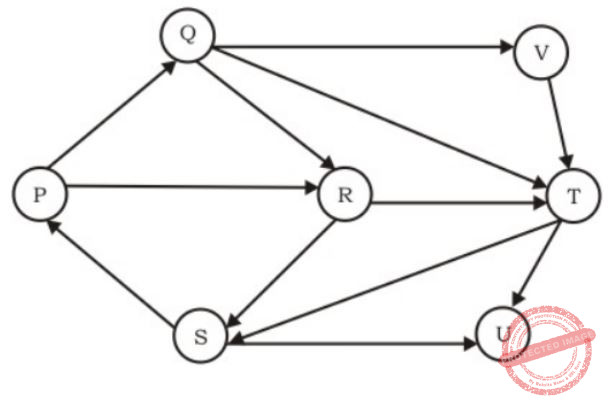A {P, Q, R, S}, {T}, {U}, {V} B {P, Q, R, S, T, V}, {U} C {P, Q, S, T, V}, {R}, {U} D {P, Q, R, S, T, U, V}
Data-Structures       Graphs
Question 46 Explanation:
In a strongly connected component every two vertices must be reachable from one to other and it is maximal component.
From given graph {P, Q, R, S, T, V} and {U} are strongly connected components.
 Question 47

Consider the depth-first-search of an undirected graph with 3 vertices P, Q, and R. Let discovery time d(u) represent the time instant when the vertex u is first visited, and finish time f(u) represent the time instant when the vertex u is last visited. Given that

```d(P) = 5 units f(P) = 12 units
d(Q) = 6 units f(Q) = 10 units
d(R) = 14 unit f(R) = 18 units ```

Which one of the following statements is TRUE about the graph?

 A There is only one connected component B There are two connected components, and P and R are connected C There are two connected components, and Q and R are connected D There are two connected components, and P and Q are connected
Data-Structures       Graphs
Question 47 Explanation:
Since, d(q) = d(p) + 1 and f(q) < f(p) which means p and q are connected and r is separate, so (D) is the answer.
 Question 48

The characters a to h have the set of frequencies based on the first 8 Fibonacci numbers as follows

`a:1, b:1, c:2, d:3, e:5, f:8, g:13, h:21`

A Huffman code is used to represent the characters. What is the sequence of characters corresponding to the following code?

`110111100111010`
 A fdheg B ecgdf C dchfg D fehdg
Algorithms        Greedy-Algorithm
Question 48 Explanation:
Huffman's tree is as follows. The two least frequently characters are taken as the children of a newly made node and the frequency of the newly made node is made equal to the sum of those two child nodes. Then the same procedure is repeated till all nodes are finished.∴ 110111100111010 = fdheg
 Question 49

Which one of the choices given below would be printed when the following program is executed?

```#include
struct test {
int i;
char *c;
}st[] = {5, "become", 4, "better", 6, "jungle", 8, "ancestor", 7, "brother"};
main ()
{
struct test *p = st;
p += 1;
++p -> c;
printf("%s,", p++ -> c);
printf("%c,", *++p -> c);
printf("%d,", p.i);
printf("%s n", p -> c);
}```
 A jungle, n, 8, ncestor B etter, u, 6, ungle C cetter, k, 6, jungle D etter, u, 8, ncestor
Programming       Programming
Question 49 Explanation:
Lets take the part of program,
Line 1 - main ( )
Line 2 - {
Line 3 - struct test *p = st;
Line 4 - p += 1;
Line 5 - ++p → c;
Line 6 - printf("%s", p++→ c);
Line 7 - printf("%c", +++p → c);
Line 8 - printf("%d", p.i);
Line 9 - printf("%s\n", p → c);
Line 10 - }
Now,
Line 3: Initially p is pointing to st, i.e., first element of st which is {5, "become"}
Line 4: Now p is pointing to {4, "better"}
Line 5: ++(p → c), since → has higher precedence, so p → c points to 'e' of "better".
Line 6: prints 'enter' and p now points to {6, "jungle"}
Line 7: ***(p → c), since → has higher precedence. So, prints 'u'.
Line 8: p → i, which is 6 so prints '6'.
Line 9: prints 'ungle' since p is pointing to 'u'.
So, output is "enter, u, 6, ungle".
 Question 50
Which one of the choices given below would be printed when the following program is executed?
```#include
void swap (int *x, int *y)
{
static int *temp;
temp = x;
x = y;
y = temp;
}
void printab ()
{
static int i, a = -3, b = -6;
i = 0;
while (i <= 4)
{
if ((i++)%2 == 1) continue;
a = a + i;
b = b + i;
}
swap (&a, &b);
printf("a =  %d, b = %d\n", a, b);
}
main()
{
printab();
printab();
}```
 A a = 0, b = 3 a = 0, b = 3 B a = 3, b = 0 a = 12, b = 9 C a = 3, b = 6 a = 3, b = 6 D a = 6, b = 3 a = 15, b = 12
Programming       Programming
Question 50 Explanation:
First of all, the swap function just swaps the pointers inside the function and has no effect on the variable being passed.
Inside print 'a' and 'b' are added to odd integers from 1 to 5, i.e., 1+3+5=9. So, in first call to print ab,
a = -3+9 = 6
b = -6+9 = 3
Static variable have one memory throughout the program run (initialized during program start) and they keep their values across function calls. So during second call to print ab,
a = 6+9 = 15
b = 3+9 = 12
 Question 51

Which one of the choices given below would be printed when the following program is executed?

```#include
int a1[] = {6, 7, 8, 18, 34, 67};
int a2[] = {23, 56, 28, 29};
int a3[] = {-12, 27, -31};
int *x[] = {a1, a2, a3};
void print(int *a[])
{
printf("%d,", a);
printf("%d,", *a);
printf("%d,", *++a);
printf("%d,", *(++a));
printf("%d/n", a[-1][+1]);
}
main()
{
print(x);
}```
 A 8, -12, 7, 23, 8 B 8, 8, 7, 23, 7 C -12, -12, 27, -31, 23 D -12, -12, 27, -31, 56
Programming       Programming
Question 51 Explanation:
1) a = *(*(a+0)+2)
It returns the value of 3rd element in a1.
First printf print 8.
2) *a = *(*(a+2))
It returns the value of 1st element in a3.
Second printf print -12.
3) *++a = *(++(*(a+0)))
a is pointing to 1st element in a1.
++a - after preincrement performed, now a is pointing to 2nd element in a1.
*++a return the value of 2nd element in a1.
Third printf print 7.
4) *(++a)
++a - after preincrement is performed 'a' is pointing to a2.
(++a) is pointing to 1st element in a2.
*(++a) returns the value of 1st element in a2.
Fourth printf print 23.
5) a[-1][+1] = *(*(a-1)+1)
(a-1) is pointing to a1.
*(a-1) is pointing to the 2nd element in a1, because in 3rd printf already a1 was incremented by 1.
*(a-1)+1 is pointing 3rd element in a1.
*(*(a-1)+1) returns the value of 3rd element in a1, i.e., 8.
 Question 52

The following function computes the value of mCn correctly for all legal values m and n (m≥1,n≥0 and m>n)

``` int func(int m, int n)
{
if (E) return 1;
else return(func(m - 1, n) + func(m - 1, n - 1));
}
```

In the above function, which of the following is the correct expression for E?

 A (n == 0) || (m == 1) B (n == 0) && (m == 1) C (n == 0) || (m == n) D (n == 0) && (m == n)
Programming       Programming
Question 52 Explanation:
We know that,
mC0 = 1
nCn = 1
 Question 53

Match the following concepts and their best possible descriptions.

Concept:
(ii) friend
(iii) constructor
(iv) protected
(v) this
(vi) inheritance
Descriptions:
A. allows to define a class to have a properties of another class
B. defining a set of similar functions
C. used in dereferncing
D. used to given a non - member function access to the private parts of body
E. a function which is automatically called when object is created
F. allows a derived class to have access to the private parts of the base
G. a pointer to the object associated with the current functions
H. used to obtain persistence
 A (i) - B, (ii) - D, (iii) - E, (iv) - F, (v) - G, (vi) - A B (i) - C, (ii) - A, (iii) - E, (iv) - D, (v) - H, (vi) - F C (i) - C, (ii) - F, (iii) - H, (iv) - A, (v) - G, (vi) - D D (i) - B, (ii) - E, (iii) - C, (iv) - F, (v) - G, (vi) - H
Programming       Match-the-Following
Question 53 Explanation:
Note: Out of syllabus.
 Question 54

The arrival time, priority, and duration of the CPU and I/O bursts for each of three processes P1, P2 and P3 are given in the table below. Each process has a CPU burst followed by an I/O burst followed by another CPU burst. Assume that each process has its own I/O resource.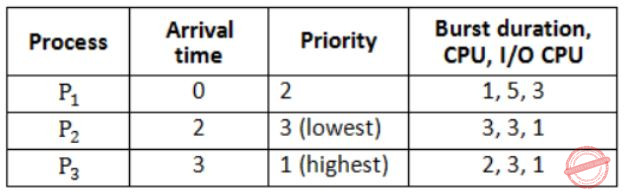The multi-programmed operating system uses preemptive priority scheduling. What are the finish times of the processes P1, P2 and P3 ?

 A 11, 15, 9 B 10, 15, 9 C 11, 16, 10 D 12, 17, 11
Operating-Systems       Process-Scheduling
Question 54 Explanation:
Gantt-chart:Hence, finish times of process.
P1 - 10
P2 - 11
P3 - 9
 Question 55

Consider the solution to the bounded buffer producer/consumer problem by using general semaphores S, F, and E. The semaphore S is the mutual exclusion semaphore initialized to 1. The semaphore F corresponds to the number of free slots in the buffer and is initialized to N. The semaphore E corresponds to the number of elements in the buffer and is initialized to 0.

```Producer Process                Consumer Process
Produce an item;                Wait(E);
Wait(F);                        Wait(S);
Wait(S);
Append the items to the buffer; Signal(S);
Signal(S);                      Signal(F);
Signal(E);                      Consume the item;  ```

Which of the following interchange operations may result in a deadlock?
1. Interchanging Wait (F) and Wait (S) in the Producer process
2. Interchanging Signal (S) and Signal (F) in the Consumer process

 A 1 only B 2 only C Neither 1 nor 2 D Both 1 and 2
Operating-Systems       Process-Synchronization
Question 55 Explanation:
Suppose Wait(F) and Wait(S) are interchanged. And let the slots are full → F=0.
Now if Wait(S) in producer succeeds, then producer will wait for Wait(F) which is never going to succeed as consumer would be waiting for Wait(S). So deadlock, can happen.
If Signal(S) and Signal(F) are interchanged in consumer, deadlock won't happen. It will just give priority to a producer compared to the next consumer waiting.
 Question 56

For each of the four processes P1, P2, P3 and P4. The total size in kilobytes (KB) and the number of segments are given below.The page size is 1 KB. The size of an entry in the page table is 4 bytes. The size of an entry in the segment table is 8 bytes. The maximum size of a segment is 256 KB. The paging method for memory management uses two-level paging, and its storage overhead is P. The storage overhead for the segmentation method is S. The storage overhead for the segmentation and paging method is T. What is the relation among the overheads for the different methods of memory management in the concurrent execution of the above four processes?

 A P < S < T B S < P < T C S < T < P D T < S < P
Operating-Systems       Virtual memory
Question 56 Explanation:
Case-1 (Two level paging):
For P1,
Page size is 1KB. So, no. of pages required for P1=195. An entry in page table is of size 4 bytes and assuming an inner level page table takes the size of a page, we can have upto 256 entries in second level page table and we require only 195 for P1. Thus only 1 second level page table is enough. So, memory overhead = 1KB (for first level) + 1KB for second level = 2KB.
For P2 and P3 also, we get 2KB each and for P4 we get 1+2 = 3KB as it requires 1 first level page table and 2 second level page tables (364 > 256). So, total overhead for their concurrent execution = 2×3+3 = 9KB. Thus P = 9KB.
Case-2 (For segmentation method):
P1 uses 4 segments → 4 entries in segment table = 4×8 = 32Bytes
Similarly, for P2, P3 and P4 we get 5×8, 3×8 and 8×8 Bytes respectively and the total overhead will be
32+40+24+64 = 160 Bytes
So, S = 160 Bytes
Case-3 (For segmentation with paging):
Here, we segment first and then page. So, we need the page table size. We are given maximum size of a segment is 256KB and page size is 1KB and thus we require 256 entries in the page table. So, total size of page table = 256 × 4 = 1024 Bytes (exactly one page size).
So, now for P1 we require 1 segment table of size 32 Bytes plus 1 page table of size 1KB.
Similarly,
P2 - 40 Bytes and 1 KB
P3 - 24 Bytes and 1 KB
P4 - 64 Bytes and 1KB
Thus, total overhead = 160 Bytes + 4KB = 4256 Bytes
So, T = 4256 Bytes
So, answer should be S < T < P.
 Question 57

The wait and signal operations of a monitor are implemented using semaphores as follows. In the following,
x is a condition variable,
mutex is a semaphore initialized to 1,
x_sem is a semaphore initialized to 0,
x_count is the number of processes waiting on semaphore x_sem, initially 0, next is a semaphore initialized to 0,
next_count is the number of processes waiting on semaphore next, initially 0.
The body of each procedure that is visible outside the monitor is replaced with the following:

```P(mutex);
⋮
body of procedure
⋮
if (next_count > 0)
V(next);
else
V(mutex); ```
Each occurrence of x.wait is replaced with the following:
```x_count = x_count + 1;
if (next_count > 0)
V(next)
else
V(mutex);
------------------------------------------------------------ E1;
x_count = x_count - 1; ```
Each occurrence of x.signal is replaced with the following:
```if (x_count > 0)
{
next_count = next_count + 1;
------------------- E2;
P(next),
next_count = next_count - 1;
} ```
For correct implementation of the monitor, statements E1 and E2 are, respectively,

 A P(x_sem), V(next) B V(next), P(x_sem) C P(next), V(x_sem) D P(x_sem), V(x_sem)
Operating-Systems       Process-Synchronization
Question 57 Explanation:
x_count is the no. of processes waiting on semaphore x_sem, initially 0.
x_count is incremented and decremented in x_wait, which shows that in between them wait(x_sem) must happen which is P(x_sem). Correspondingly V(x_sem) must happen in x_signal. So, D choice.
 Question 58

A software program consists of two modules M1 and M2 that can fail independently, but never simultaneously. The program is considered to have failed if any of these modules fails. Both the modules are ‘repairable’ and so the program starts working again as soon as the repair is done. Assume that the mean time to failure (MTTF) of M1is T1 with a mean time to repair (MTTR) of R1. The MTTF of M2 is T2 with an MTTR of R2. What is the availability of the overall program given that the failure and repair times are all exponentially distributed random variables?

 A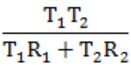BCDQuestion 58 Explanation:
Note: Out of syllabus.
 Question 59

Consider the following structure chart diagram. The boxes have function names embedded in them, while the variables are indicated along the arcs.Given below are a set of statements relevant to the above diagram.
I. F3 and F6 can be in the same module.
II. F4 and F6 can be in the same module.
III. A4 is both an output and a control variable.
IV. It is incorrect to pass A1 as data and use it as a control variable.
Which combination of these statements is TRUE?

 A III and IV B I and IV C II and IV D I, II and IV
Question 59 Explanation:
Note: Out of syllabus.
 Question 60

Consider a relation R with five attributes V, W, X, Y, and Z. The following functional dependencies hold: VY → W, WX → Z, and ZY → V.
Which of the following is a candidate key for R?

 A VXZ B VXY C VWXY D VWXYZ
Database-Management-System       Functional-Dependency
Question 60 Explanation:
As we can see that attribute XY do not appear in RHS of an FD, they need to be a part of key.
Candidate keys are
VXY, WXY, ZXY
 Question 61

In a database file structure, the search key field is 9 bytes long, the block size is 512 bytes, a record pointer is 7 bytes and a block pointer is 6 bytes. The largest possible order of a non-leaf node in a B+ tree implementing this file structure is

 A 23 B 24 C 34 D 44
Database-Management-System       B+-Trees
Question 61 Explanation:
From the structure of B+ tree we can get this question:
n × p + (n - 1) × k ≤ B (for non-leaf node)
Here, n = order, p = tree/block/index pointer, B = size of block
So,
n × p + (n - 1) × k ≤ B
n × 6 + (n - 1) × 9 ≤ 512
n ≤ 34.77
∴ n = 34
 Question 62

Consider the following XML DTD describing course information in a university:

```<!ELEMENT Univ (Course+, Prof+)>
<!ELEMENT Course (Title, Eval*)>
<!ATTLIST Course Number ID #REQUIRED Instructor IDREF #IMPLIED>
<!ELEMENT Title (#PCDATA)>
<!ELEMENT Eval (#PCDATA)>
<!ATTLIST Eval Score CDATA #REQUIRED>
<!ELEMENT Prof EMPTY>
<!ATTLIST Prof Name ID #REQUIRED Teaches IDREF #IMPLIED>
```

What is returned by the following XQuery?

```let \$as := //@Score
for \$c in /Univ/Course[Eval]
let \$cs := \$c/Eval?@Score
where min(\$cs) > avg(\$as)
return \$c ```
 A The professor with the lowest course evaluation B Professors who have all their course evaluations above the university average C The course with the lowest evaluation D Courses with all evaluations above the university average
Question 62 Explanation:
Note: Out of syllabus.
 Question 63

A router uses the following routing table: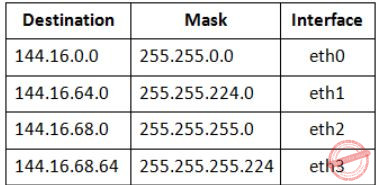A packet bearing a destination address 144.16.68.117 arrives at the router. On which interface will it be forwarded?

 A eth0 B eth1 C eth2 D eth3
Question 63 Explanation:
144.16.68.117 = 144.16.68.01110101 AND 255.255.255.224 = 255.255.255.11100000
= 144.16.68.96(Not matching with destination)
Now, take 255.255.255.0
144.16.68.117 AND 255.255.255.0
= 144.16.68.0 (matched)
Hence, option (C) is correct.
 Question 64

Suppose that it takes 1 unit of time to transmit a packet (of fixed size) on a communication link. The link layer uses a window flow control protocol with a window size of N packets. Each packet causes an ack or a nak to be generated by the receiver, and ack/nak transmission times are negligible. Further, the round trip time on the link is equal to N units. Consider time i > N. If only acks have been received till time i(no naks), then the goodput evaluated at the transmitter at time i(in packets per unit time) is

 A 1 – N/i B i/(N + i) C 1 D 1 – e(i/N)
Computer-Networks       Sliding-Window-Protocol
Question 64 Explanation:
Goodput is the application level throughout, i.e., the no. of useful information bits delivered by the network to a certain destination per unit of time.
So, successful delivery of packet can be assured if ack has been received for it.
So till time 'i' we would have transmitted 'i' packets but only (i - N) can be acknowledged as minimum time for a packet to get acknowledged is N (since RTT is N which is equal to the window size, there is no waiting for the sender).
So, successfully delivered packets = (i - N)
Time for transmission = i
Goodput = Successfully delivered data/Time
= (i - N)/i
= 1 - N/i
 Question 65

In the 4B/5B encoding scheme, every 4 bits of data are encoded in a 5-bit codeword. It is required that the codewords have at most 1 leading and at most 1 trailing zero. How many such codewords are possible?

 A 14 B 16 C 18 D 20
Computer-Networks       Encoding
Question 65 Explanation:
It says we have 5 bit codeword such that "it can't have two consecutive zeros in first and second bit" and also "can't have two consecutive zeros in last two bits".
Codeword with first two bits '0'
= 0 0 x x x
= 23
= 8
Codeword with last two bits '0'
= x x x 0 0
= 23 = 8
Codeword with first two and last two bits '0'
= 0 0 x 0 0
= 2
Codeword with first or last two bits '0'
= 8 + 8 - 2
= 14
Therefore possible codewords
= 32 - 14
= 18
 Question 66

A router has two full-duplex Ethernet interfaces each operating at 100 Mb/s. Ethernet frames are at least 84 bytes long (including the Preamble and the Inter-Packet-Gap). The maximum packet processing time at the router for wirespeed forwarding to be possible is (in micro­seconds)

 A 0.01 B 3.36 C 6.72 D 8
Computer-Networks       Ethernet
Question 66 Explanation:
Let's first calculate transmission time Tt,
Tt = 84×8/10×106 = 6.72μs
But since a router has two full-duplex ethernet interfaces, so the maximum processing time should be,
6.72/2 μs = 3.36μs
 Question 67

A link of capacity 100 Mbps is carrying traffic from a number of sources. Each source generates an on-off traffic stream; when the source is on, the rate of traffic is 10 Mbps, and when the source is off, the rate of traffic is zero. The duty cycle, which is the ratio of on-time to off-time, is 1:2. When there is no buffer at the link, the minimum number of sources that can be multiplexed on the link so that link capacity is not wasted and no data loss occurs is S1. Assuming that all sources are synchronized and that the link is provided with a large buffer, the maximum number of sources that can be multiplexed so that no data loss occurs is S2. The values of S1 and S2 are, respectively,

 A 10 and 30 B 12 and 25 C 5 and 33 D 15 and 22
Computer-Networks       Network-Layer
Question 67 Explanation:
For S1,
Since there is no buffer and constraint given is there should not be any data lost, and no wastage of capacity as well.
Since data should not be lost, we calculate for the extreme case when all sources are ontime (that is transmitting).
10Mbps × n-station ≤ 100Mbps
n = 10 = S1
In the next part of question, it is given that the link is provided with large buffer and we are asked to find out large no. of stations.
For that we calculate expected value of bandwidth usage,
E = 1/3 × 10 + 1/3 × 10 + .......+ ....... n-station times ≤ 100Mbps
⇒ 1/3 × 10 × n-station ≤ 100Mbps ⇒ n-station = 30 = S2
 Question 68

On a wireless link, the probability of packet error is 0.2. A stop-and-wait protocol is used to transfer data across the link. The channel condition is assumed to be independent from transmission to transmission. What is the average number of transmission attempts required to transfer 100 packets?

 A 100 B 125 C 150 D 200
Computer-Networks       Stop-and-Wait-protocol
Question 68 Explanation:
Total no. of re-transmissions for one frame, in general, is 1/(1-P) where P is probability of error.
So here it would be for one frame = 1/(1-0.2) = 1/0.8
So for 100 frames = 100/0.8 = 125
 Question 69

A program on machine X attempts to open a UDP connection to port 5376 on a machine Y, and a TCP connection to port 8632 on machine Z. However, there are no applications listening at the corresponding ports on Y and Z. An ICMP Port Unreachable error will be generated by

 A Y but not Z B Z but not Y C Neither Y nor Z D Both Y and Z
Computer-Networks       TCP-and-UDP
Question 69 Explanation:
When an IP packet is lost or discarded ICMP packet will be generated by receiver or the router. It doesn't matter whether its containing TCP or UDP inside it.
 Question 70

 A is necessarily 255.255.224.0 B is necessarily 255.255.240.0 C is necessarily 255.255.248.0 D could be any one of 255.255.224.0, 255.255.240.0, 255.255.248.0
Question 70 Explanation:
In the broadcast address for a subnet, all the host bits are set to 1. So as long as all the bits to the right are 1, bits left to it can be taken as possible subnet.
.95.255 or .01011111.11111111
(as in class B, 16 bits each are used for network and host)
So, we can take minimum 3 bits (from left) as subnet and make rest as host bits (as they are 1)
.224.0 → 11100000.00000000 (leftmost 3 bits for subnet)
.240.0 → 11110000.00000000 (leftmost 4 bits for subnet)
.248.0 → 11111000.00000000 (leftmost 5 bits for subnet)
 Question 71

An array X of n distinct integers is interpreted as a complete binary tree. The index of the first element of the array is 0. The index of the parent of element X[i],i≠0 is?

 A ⌊i/2⌋ B ⌈(i-1)/2⌉ C ⌈i/2⌉ D ⌈i/2⌉ - 1
Data-Structures       Binary-Trees
Question 71 Explanation:
If index of array start with 1 then directly divide the ith value by 2 and take floor. If index start with '0' then ⌈i/2⌉ - 1.
 Question 72

An array X of n distinct integers is interpreted as a complete binary tree. The index of the first element of the array is 0. If only the root node does not satisfy the heap property, the algorithm to convert the complete binary tree into a heap has the best asymptotic time complexity of

 A O(n) B O(log n) C O(n log n) D O(n log log n)
Data-Structures       Binary-Trees
Question 72 Explanation:
It takes O(log n) to heapify an element of heap.
 Question 73

An array X of n distinct integers is interpreted as a complete binary tree. The index of the first element of the array is 0. If the root node is at level 0, the level of element X[i], i ≠ 0, is

 A ⌊log2 i⌋ B ⌈log2 (i + 1)⌉ C ⌊log2 (i + 1)⌋ D ⌈log2 i⌉
Data-Structures       Binary-Trees
Question 73 Explanation:
If the root node is at level 0 then the level of element X[i] is ⌊log2 (i + 1)⌋.
 Question 74

Consider the following program module:

```void swap(float* A1, float* A2)
{
float temp;
if (*A1 = = *A2) return;
temp = *A1;
*A1 = *A2;
*A2 = temp;
return;
} ```

The program volume for the above module using Halstead's method is

 A 60 B 63 C 66 D 69
Question 74 Explanation:
Note: Out of syllabus.
 Question 75

Consider the following program module:

```void swap(float* A1, float* A2)
{
float temp;
if (*A1 = = *A2) return;
temp = *A1;
*A1 = *A2;
*A2 = temp;
return;
} ```

The program effort for the above module using Halstead's method is

 A 315 B 330 C 393 D 403
Question 75 Explanation:
Note: Out of syllabus.
 Question 76

Consider the following set of linear operations:
x + y/2 = 9
3x + y = 10
The value of the Frobenius norm for the above system of equations is:

 A 0.5 B 0.75 C 1.5 D 2
Engineering-Mathematics       Linear-Algebra
Question 76 Explanation: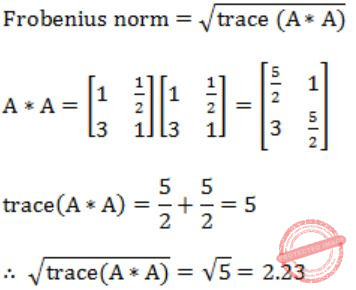Question 77

Consider the following set of linear operations:
x + y/2 = 9
3x + y = 10
What can be said about the Gauss-Siedel iterative method for solving the above set of linear equations?

 A it will converge B it will diverse C it will neither converge nor diverse D It is not applicable
Engineering-Mathematics       Linear-Algebra
Question 77 Explanation:
As,
|1| + |1/2| <= |9|
and |3| + |1| <= |10|
 Question 78

A pipelined processor uses a 4-stage instruction pipeline with the following stages: Instruction fetch (IF), Instruction decode (ID), Execute (EX) and Writeback (WB). The arithmetic operations as well as the load and store operations are carried out in the EX stage. The sequence of instructions corresponding to the statement X = (S - R * (P + Q))/T is given below. The values of variables P, Q, R, S and T are available in the registers R0, R1, R2, R3 and R4 respectively, before the execution of the instruction sequence.The number of Read-After-Write (RAW) dependencies, Write-After-Read( WAR) dependencies, and Write-After-Write (WAW) dependencies in the sequence of instructions are, respectively,

 A 2, 2, 4 B 3, 2, 3 C 4, 2, 2 D 3, 3, 2
Computer-Organization       Pipelining
Question 78 Explanation:
RAW:
I1 - I2 (R5)
I2 - I3 (R6)
I3 - I4 (R5)
I4 - I5 (R6)
WAR:
I2 - I3 (R5)
I3 - I4 (R6)
WAW:
I1 - I3 (R5)
I3 - I4 (R6)
 Question 79

A pipelined processor uses a 4-stage instruction pipeline with the following stages: Instruction fetch (IF), Instruction decode (ID), Execute (EX) and Writeback (WB). The arithmetic operations as well as the load and store operations are carried out in the EX stage. The sequence of instructions corresponding to the statement X = (S - R * (P + Q))/T is given below. The values of variables P, Q, R, S and T are available in the registers R0, R1, R2, R3 and R4 respectively, before the execution of the instruction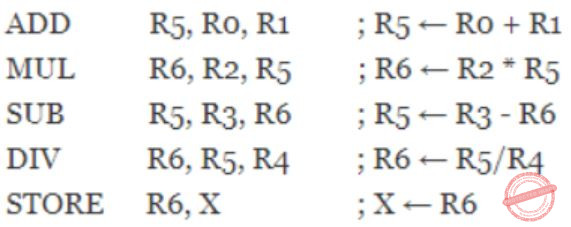The IF, ID and WB stages take 1 clock cycle each. The EX stage takes 1 clock cycle each for the ADD, SUB and STORE operations, and 3 clock cycles each for MUL and DIV operations. Operand forwarding from the EX stage to the ID stage is used. The number of clock cycles required to complete the sequence of instructions is

 A 10 B 12 C 14 D 16
Computer-Organization       Pipelining
Question 79 Explanation: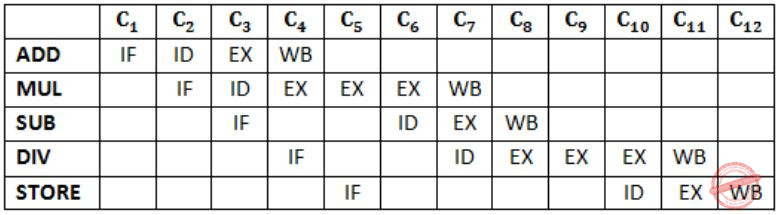Question 80

Let L be a regular language. Consider the constructions on L below:
I. repeat (L) = {ww | w ∊ L}
II. prefix (L) = {u | ∃v : uv ∊ L}
III. suffix (L) = {v | ∃u : uv ∊ L}
IV. half (L) = {u | ∃v : | v | = | u | and uv ∊ L}
Which of the constructions could lead to a non-regular language?

 A Both I and IV B Only I C Only IV D Both II and III
Theory-of-Computation       Regular-Language
Question 80 Explanation:
Repeat(L) = {ww|w ∈ L} is non-regular language.
Half (L), Suffix (L) and Prefix (L) are regular languages.
 Question 81

Let L be a regular language. Consider the constructions on L below:
(I) repeat (L) = {ww | w ∊ L}
(II) prefix (L) = {u | ∃v : uv ∊ L}
(III) suffix (L) = {v | ∃u uv ∊ L}
(IV) half (L) = {u | ∃v : | v | = | u | and uv ∊ L}
Which choice of L is best suited to support your answer above?

 A (a + b)* B {ϵ, a, ab, bab} C (ab)* D {anbn | n ≥ 0}
Theory-of-Computation       Regular-Language
Question 81 Explanation:
A counter example which proves all the conclusions of the last question in one go should have the following properties:
1) L should be regular due to demand of question.
2) L should be an infinite set of strings.
3) L should have more than one alphabet in its grammar, otherwise repeat(L) would be regular.
∴ (a + b)* is the perfect example to support the conclusions of last questions.
 Question 82

A software project has four phases P1, P2, P3 and P4. Of these phases, P1 Is the first one and needs to be completed before any other phase can commence. Phases P2 and P3 can be executed in parallel. Phase P4 cannot commence until both P2 and P3 are completed. The optimistic, most likely, and pessimistic estimates of the phase completion times in days, for Pl, P2, P3 and P4 are, respectively, (11, 15, 25), (7, 8, 15), (8, 9, 22), and (3, 8, 19).
The critical path for the above project and the slack of P2 are, respectively,

 A P1-P2-P4, 1 day B P1-P3-P4, 1 day C P1-P3-P4, 2 days D P1-P2-P4, 2 days
Question 82 Explanation:
Note: Out of syllabus.
 Question 83

A software project has four phases P1, P2, P3 and P4. Of these phases, P1 Is the first one and needs to be completed before any other phase can commence. Phases P2 and P3 can be executed in parallel. Phase P4 cannot commence until both P2 and P3 are completed. The optimistic, most likely, and pessimistic estimates of the phase completion times in days, for Pl, P2, P3 and P4 are, respectively, (11, 15, 25), (7, 8, 15), (8, 9, 22), and (3, 8, 19).
The costs (in Rupees per day) of crashing the expected phase completion times for the four phases, respectively, are 100, 2000, 50, and 1000. Assume that the expected phase completion times of the phases cannot be crashed below their respective most likely completion times. The minimum and the maximum amounts (in Rupees) that can be spent on crashing so that ALL paths are critical are, respectively.

 A 100 and 1000 B 100 and 1200 C 150 and 1200 D 200 and 2000
Question 83 Explanation:
Note: Out of syllabus.
 Question 84

Consider a database with three relation instances shown below. The primary keys for the Drivers and Cars relation are did and cid respectively and the records are stored in ascending order of these primary keys as given in the tables. No indexing is availableWhat is the output of the following SQL query?

```select D.dname
from Drivers D
where D.did in (
select R.did
from Cars C, Reserves R
where R.cid = C.cid and C.colour = 'red'
intersect
select R.did
from Cars C, Reserves R
where R.cid = C.cid and C.colour = 'green'
)```
 A Karthikeyan, Boris B Sachin, Salman C Karthikeyan, Boris, Sachin D Schumacher, Senna
Database-Management-System       SQL
Question 84 Explanation:
For colour = "Red"
did = {22, 22, 31, 31, 64}
For colour = "Green"
did = {22, 31, 74}
Intersection of Red and Green will be = {22, 31}, which is Karthikeyan and Boris.
 Question 85

Consider a database with three relation instances shown below. The primary keys for the Drivers and Cars relation are did and cid respectively and the records are stored in ascending order of these primary keys as given in the tables. No indexing is availableLet n be the number of comparisons performed when the above SQL query is optimally executed. If linear search is used to locate a tuple in a relation using primary key, then n lies in the range

 A 36 - 40 B 44 - 48 C 60 - 64 D 100 - 104
Database-Management-System       SQL
Question 85 Explanation:
(4) for taking red cars with (20) comparisons for did and (4) for finding green cars with (10) for did.
red did : 22, 31, 64
green did : 22, 31, 74
(6) for intersection
(1) for searching 22 in driver relation, and (3) for searching 31.
Total: 38 + 6 + 4 = 48
There are 85 questions to complete.